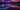## 什么是闭包

function a() {
let i = 0
console.log(i)
function b() {
i++
console.log(i)
}
return b
}

let fb = a()
fb()
// 0
// 1

let fb = a()
// a函数中声明变量i = 0，并且将i的值打印出来
// a函数中声明b函数，在b函数中将i的值增加1
// 将b函数返回，a函数执行完毕
fb()
// i的值增加1，并将其打印出来

## 防抖函数

let lock = false

function submit() {
if (!lock) {
lock = true
setTimeout(() => {
console.log('提交完毕')
lock = false
}, 100) // 100毫秒内不允许再次执行
}
}
function invoke() {
let i = 0
const timer = setInterval(() => {
if (i > 1) {
clearInterval(timer)
return
}
submit()
i++
}, 60)
}

invoke()
// 提交完毕

function debounce(func, delay) {
let timer
let lock = false
return function(params) {
if (lock) {
// 触发间隔未结束，重新设定计时器
clearTimeout(timer)
timer = setTimeout(() => {
lock = false
clearTimeout(timer)
timer = null
}, delay)
} else {
lock = true
func(params)
}
}
}

function submit() {
console.log('提交完毕')
}

const debouncedSubmit = debounce(submit, 100)

function invoke() {
let i = 0
const timer = setInterval(() => {
if (i > 1) {
clearInterval(timer)
return
}
debouncedSubmit()
i++
}, 100)
}

invoke()
// 提交完毕

function debounce(func, delay, immediate = true) {
let timer
let lock = !immediate
return function(params) {
if (lock) {
// 触发间隔未结束，重新设定计时器
clearTimeout(timer)
timer = setTimeout(() => {
lock = false
clearTimeout(timer)
timer = null
if (!immediate) {
func(params)
}
}, delay)
} else {
lock = true
func(params)
}
}
}

## 思考Buy me a cup of coffee ☕.